# Improve Magnetic Card Reading in the Presence of Noise

### Abstract

Most magnetic read head data sheets do not fully specify the frequency-dependent components and are often vague when specifying other key parameters. In some cases, the specifications of two very similar heads from two different manufacturers might be quite different in terms of parameters specified and omitted. The limitations in the data sheets make designing an optimum card reading system unnecessarily difficult and time consuming. This document outlines a strategy to overcome the above shortcomings and offers guidelines to overcome the noise issues.

### Introduction

One of the most crucial parts of any magnetic card reader (MCR) system is the magnetic read head (MRH). When a card is swiped, the MRH converts the stored data in the card's magnetic stripe to a voltage. Other MCR blocks process the converted voltage to extract the stored data. Large differences in magnetic field strengths from one card to another and differences in swipe speeds from one person to another make designing an MCR not a trivial task.

Adding to the MCR design difficulty are the MRH data sheets, which do not fully specify the frequency-dependent components and are often vague when specifying other key parameters. In some cases the data-sheet specifications of two very similar heads from two different manufacturers differ significantly in the list of parameters specified and those omitted. These differences are especially troublesome and disconcerting when you are trying to minimize noise issues in an MCR system. They certainly make designing an optimum card-reading system unnecessarily difficult and time-consuming. This article outlines a strategy to resolve these specification issues, and then explains how to optimize and overcome the noise issues in an MCR using a secure microcontroller optimized for the task.

### Magnetic-Stripe Card Basics

Figure 1 shows a magnetic stripe card with three tracks. Several ISO/IEC¹ standards define important card properties such as the physical size, exact location of the stripes, magnetic properties, and magnetic track data structures. Track 1 standards were created by the International Air Transportation Association (IATA). Track 2 standards were created by the banking industry (American Bankers Association, ABA), and Track 3 standards were created by the thrift-savings industry.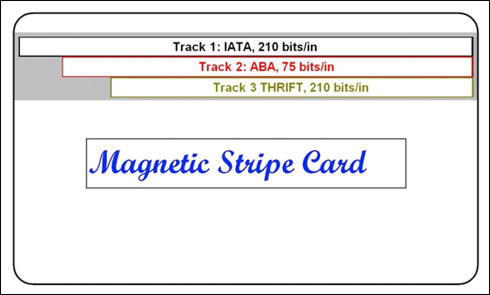A two-frequency coherent phase (F2F) technique is used for encoding the data on magnetic stripe cards. As shown in Figure 2, the binary data is encoded along the track by magnetizing stripe areas with different polarities. The polarity of the transitions is arbitrary, since only the relative space between the transitions implies a binary 1 or a binary 0.A binary 0 is encoded with a two-unit bar magnet, while a pair of one-unit bars represents a binary 1. Each bit occupies the same physical length on the stripe. A bit with an additional flux transition in the middle of its length is a binary 1.

The spectrum of a continuous signal with F2F coding contains two fundamental frequencies, f0 and f1, where f0 is the fundamental of the square wave for binary 0, and f1 = 2f0 is the fundamental of the square wave for binary 1. Hence, the name F2F. The average amplitude of the binary 0 waveform is twice that of the binary 1 waveform, A0 = 2A1.

Figure 3 shows the combined spectrum of F2F encoded binary 0s and 1s normalized to f0. Note that most of the signal energy resides between f0 and 3f0. Thus, to get a good approximation of a rectangular waveform containing a series of F2F encoded binary 1s and 0s, it is enough to recover the two fundamentals (f0 and f1) and the 3rd harmonic of f0.Due to varying binary patterns, moreover, there will be other components below f0. However, we can see from Fourier analysis that the amplitudes of these components decrease very quickly for decreasing frequencies. Thus, a bandwidth from 0.5f0 to 3f0 is adequate for recovering an F2F-encoded rectangular waveform.²

To estimate the minimum and maximum bit rates for f0 and f1, we need to know the swipe speed range and the track recording density. From ISO/IEC standards, the recording density of Tracks 1 and 3 is 210 bits/in (8.27 bits/mm), while that of Track 2 is 75 bits/in (2.95 bits/mm).

For calculating f0,min we take the slowest swipe speed supported and multiply it by the Track 2 density numbers. For f1,max we pick the fastest swipe speed supported and multiply it by the density of Tracks 1 or 3.

For target swipe rates of 2in/s to 100in/s (5cm/s to 254cm/s), the range of f0 and f1 values is calculated as follows:

• Tracks 1 and 3: f0,min = 0.42kbps and f1,max = 42kbps
• Track 2: f0,min = 0.15kbps and f1,max = 15kbps

The importance of knowing f0,min and f1,max will become clear once we have the MRH model and study its transfer function.

Swiping a magnetic stripe card past a stationary MRH results in a changing magnetic flux that produces a moving electric field. Thus, a voltage is induced at the MRH's output. Figure 2 can be used to study the reading process. Starting from the top, a magnetic stripe moving past an MRH results in changing flux events that induce a voltage at the MRH's output terminals. The open-circuit readback voltage without any electrical losses is given by the well-known expression:³Where:
E() = open-circuit voltage
K = a constant relating the effects of magnetic-stripe velocity, head width, and the number of coil turns in the MRH
M[(x -), y] = magnetic-stripe material magnetization
y1 = spacing from the head to the top of the magnetic-stripe
y2 = spacing from the head to the bottom of the magnetic-stripe

Clearly, the above equation is highly complicated and not very intuitive for circuit analysis and design. We can use the basic principles to determine a model as follows.

The MRH transfers magnetic energy to electric energy. Since the MRH's input is a changing magnetic field and its output is a changing electric field, the model should contain at least one inductive element, Lh, and one capacitive element, Ch. In real systems some energy is always spent during the transformation. Thus, the model must also contain a resistive element, Rh. In practice, an MRH will not only have Ch across its two terminals, but it will also have an external impedance, Zo, from, for example, connecting wires, PCB traces, IC pins, probes, etc.

A model corresponding to the nth harmonic frequency, fn, is shown in Figure 4a, which then is simplified as Figure 4b.3, 4, 5 The transfer function of the 2nd-order circuit in Figure 4b is easily calculated as: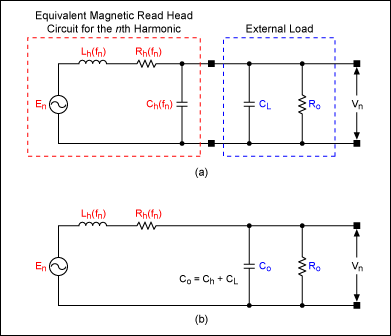Note that the above transfer function does not contain any mechanical or magnetic terms, e.g., the swipe speed, head geometry, head and stripe separation, and the stripe magnetic properties. Thus, the transfer function is more intuitive for circuit design.

To further simplify things, we propose using a lumped model characterized at the highest system frequency instead of limiting the model to the swipe speeds.

Next we compare the electrical specifications of MRHs from some leading manufacturers. Table 1 lists the key specifications that are needed for the model of Figure 4b. Notice the different amount of detail. While both Manufacturers A and C specify several electrical parameters, Manufacturer B specifies only one: peak-to-peak head readout level.

 Parameter Manufacturer A Manufacturer B Manufacturer C VO(P-P) (mV) 20 19 35 Lh (mH) 25 — 110 Ch (pF) — — — Rh (Ω) 110 — 280

The following questions may arise about the missing information.

• Head Inductance (Lh). How does Lh behave over a larger frequency range? How does Lh behave when carrying currents other than what is specified?
• Head DC Resistance (Rh). What voltage level is applied across the head terminals?
• Head Read Output Level (VO(P-P)). What type of test card is used? What is the card swipe speed? What is the load across the head?
• Head Capacitance (Ch). What is the capacitance between the two head terminals? Does it change with frequency?

To appreciate the importance of the above parameters, we examine the transfer function's denominator and find its roots by setting it to zero: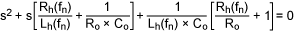To keep expressions simple and better understand the 2nd-order behavior, several network analysis books6, 7 use standard forms to write equations. One standard uses the form:

s² + 2α × s + ωo² = 0

Whose roots are: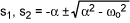Where:
α is the damping attenuation:ωo is the resonance frequency:Therefore, depending on the values of α and ωo, the roots of the natural response can be real, complex, or imaginary. For readers who are familiar with other standard forms, we now define the damping factor as ζ = αo (note: quality factor Q = 1/2ζ) and use the other standard form:

s² + 2ζωo × s + ωo² = 0

Whose roots are: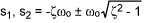When a nonzero forcing function (such as a step, a ramp, or an impulse) is applied to the system, the location of the roots in the s-plane directly affects the settling behavior. Figure 5 shows the settling behavior for various ζ values when a step is applied at t = 0. Specifically, the settling behavior is categorized as follows:

ζ > 1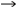overdamped
ζ < 1underdamped
ζ = 1critically damped
ζ = 0undamped or oscillatory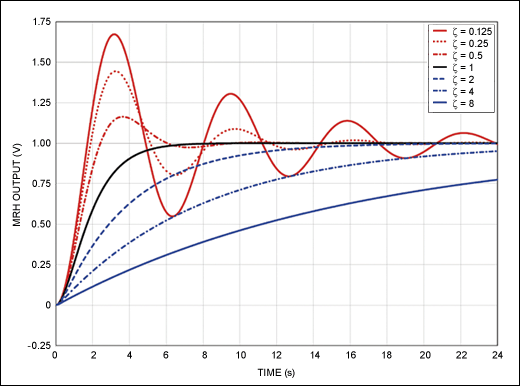From Figure 5 we observe that in an underdamped system ringing occurs, which can cause reading errors due to false peaks and false zero crossings. However, if the system is drastically overdamped, timing errors can occur from slow settling and reading errors can occur from shifts in the peaks. After analyzing an MRH's time-domain behavior, we next look at its frequency-domain behavior.

Figure 6 shows the frequency response of the transfer function, Tn(s), which is normalized to its resonance frequency, ωo. We observe peaking as we approach the system resonance frequency. This is due to the intrinsic nature of the circuit shown in Figure 4b, i.e., a parallel RLC. Depending on the swipe speed, this peaking can also cause reading errors.Recall that when trying to recover F2F-encoded binary data, we need the two fundamental frequencies, f0 and f1, and at least the 3rd harmonic of f0. From Figure 3 we see that most of the signal energy is in the vicinity of 0.5f0 to 3.5f0, while a small portion is around 6f0.

What will happen to the recovered F2F waveform if higher harmonics were gained up? From Fourier analysis we know that the recovered waveform shape will change as the magnitudes of the higher harmonic coefficients change. Thus, for some cases the gain peaking shown in Figure 5 can reach a point where the 3rd and the 6th harmonics are amplified to such a level that the recovered signal is severely distorted. Any distortion that results in false peaks and zero crossings will lead to reading errors.

The above point is emphasized in Figure 7, which mathematically shows MRH output voltage for two different gains at higher harmonics: solid blue is the unity gain and red for a gain of two. The dotted black lines are the ZX hysteresis limits. Clearly, as the gain doubles, the MRH output signal in red shows more distortion, false peaks, and zero crossings.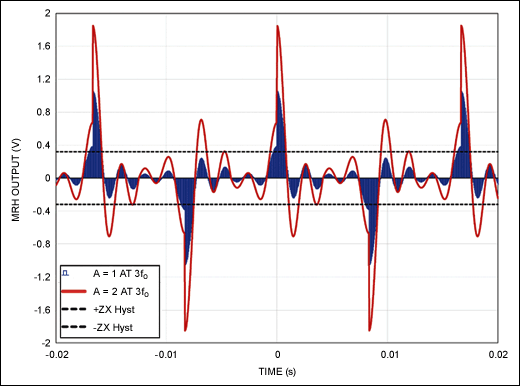Furthermore, the MRH will not only experience disturbances due to varying card-swipe speeds. It will also see disturbances at much higher frequencies that might be present in the overall system, for example, a high-frequency system clock. Because of gain peaking, this too can result in signal distortion and possible reading errors. Thus, for designing an optimum card-reading system, it is crucial to know the frequency behavior of the MRH beyond the swipe rates. One must characterize the MRH frequency behavior at least to the highest system frequency.

We used a commercially available impedance/gain-phase analyzer to find the equivalent circuits of several MRHs from different manufacturers. The characterization included single-, double-, and triple-track MRHs that were used in the MCR based on the DeepCover® Secure Microcontroller (MAXQ1740). As 12MHz is the maximum system clock frequency for the MAXQ1740, each MRH was characterized from 100Hz to 12MHz (100Hz is analyzer's limit). Table 2 shows parameter averages for triple-track MRHs.

 Parameter MRH 1 MRH 2 MRH 3 MRH 4 Lh (mH) 13.67 58.09 13.20 57.43 Ch (pF) 22.15 31.11 20.60 16.97 Rh (Ω) 146.78 234.57 145.72 214.51

### Analyzing the Measured Parameters

Comparing the parameters in Table 2, we see that MRH 1 and MRH 3 are very similar. The relative differences between their parameters are: ΔLh ~ 3.6%, ΔRh ~ 0.7%, and ΔCh ~ 7.5%. For MRH 2 and MRH 4, the relative differences in their parameters are: ΔLh ~ 1.2%, ΔRh ~ 9.4%, and ΔCh ~ 83%. Since Ch affects both α and ωo, for similar conditions we can expect the behavior of MRHs 1 and 3 to be very similar. We can expect the behavior of MRHs 2 and 4 to track below their resonance frequencies, but then change as the frequencies reach close to, and beyond, their respective resonance points.

The last two points become obvious when we plot the transfer function's frequency response for the characterized MRHs as shown in Figure 8. The load in Figure 8 is 1G that results in a damping ratio of 0.03. The plots for MRHs 1 and 3 are virtually the same, while the plots for MRHs 2 and 4 show increasing differences around the resonance frequencies. The increase in magnitude could result in reading errors, as described earlier.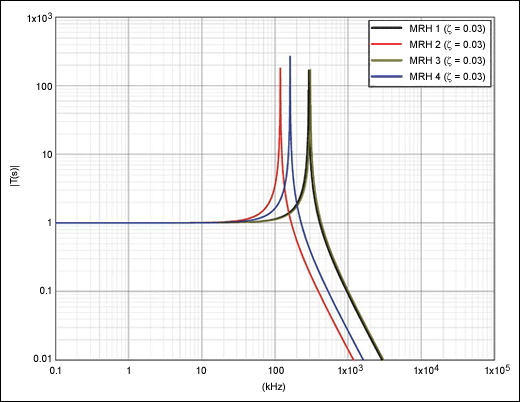Figure 9 shows MRH transfer functions for the frequency range of 150kHz to 300kHz, i.e., 3rd and 6th harmonics corresponding to the maximum card-swipe rate of 100in/s (254cm/s). We can see that as the swipe rates increase, so do the MRH transfer-function magnitude values. The main concern here is that if higher harmonics are gained up beyond a point, false zero crossings and peaks can occur as shown in Figure 7. Also, if signals larger than the maximum allowed appear at the interface between the head and the card reader's inputs, then reading errors might occur.

Two factors cause a larger signal. First, a faster swipe increases the rate of magnetic flux change. This, according to Faraday's law, induces a larger inductor voltage, resulting in a larger inductor current. Second, a larger current flowing through a larger impedance results in a larger output voltage in accordance with Ohm's law.

Within the card swipe-rate range we need to limit the peaking to less than or equal to 20, which is the ratio between the maximum and minimum gains of the MAXQ1740 magnetic card reader. Figure 9 shows that the impedance change for MRHs 1 and 3 is less than 20, but the change for MRH 2 and MRH 4 is nearly 30 and exceeds the limit of 20.What will happen if a damping resistor is added at the output of the MRH? Figure 10 shows the transfer function plots for three arbitrarily different external loads values: 100kΩ, 10kΩ, and 1kΩ. We see in Figure 10 that for lower-value external resistors, the peaking is reduced compared to that shown in Figure 9. Note that for 1kΩ loads the gain at the 3rd harmonic is severely reduced for all four MRHs. This can be a problem. With 100k loads, for MRHs 2 and 3, the gains peaks at the 3rd harmonic, while for MRHs 2 and 3, the gain peaks at the 6th harmonic. The main point here is that we cannot arbitrarily pick the Ro values.When using external resistor Ro across the MRH terminals, it is very important to ensure that the damping ratio, ζ, stays as close to the unity as possible. Figure 11 plots ζ vs. Ro for the four characterized MRHs. For ζ = 1, we need Ro ≈ 12kΩ for both MRHs 1 and 3; Ro ≈ 22kΩ for MRH 2; and Ro ≈ 28kΩ for MRH 4. Figure 12 shows that transfer function with optimum load values. Comparing Figure 12 to Figure 10, we note that the gain does not peaking at the 3rd harmonic and stays close to unity.While the maximum Ro is set by ζ = 1, the minimum Ro value depends on the minimum signal supported and the head DC resistance, Rh. As a general rule, keep Ro ≥ 5Rh so that Ro in parallel with Rh will not attenuate the head output signal by more than 20%.

There are several key points to remember here. First, due to parallel RLC, the transfer function peaks around the resonance frequency, ωo. Therefore, limit this peaking for the range corresponding to the 3rd and the 6th harmonics of card swipe rate, e.g., 150kHz to 300kHz for the card swipe-rate range of 42kHz to 50kHz. Second, the system behavior can be adjusted by placing Ro across the head reader's terminals. Changing Ro changes the damping ratio, ζ. Finally, select Ro value to make the system critically damped and let the lead wiring and PCB routing set the Co value.

With a method now to resolve the discrepancies in MRH specifications, we can improve card reading performance. Our focus will be on reducing the effects of noise which mostly affect the zero crossings (ZX). After characterizing the MRH model for the entire frequency range (note: the characterization must include lead wires and the PCB routing), we follow these steps.

Step 1. Choose an Ro value to get an appropriate damping ratio and limit the gain peaking.

• In general, the target should be a critically damped to slightly overdamped system. As an exception, if for some cases the gain at the 3rd harmonic drops below half, then we can equalize the gain by a slightly underdamped system.
• An underdamped system can introduce noise from ringing of the input signal. Ringing noise adversely affects the ZX, but may also result in false peaks due to gain peaking.
• Keep Ro ≥ 5Rh with the maximum Ro set by ζ = 1.

Step 2. On noisier printed circuit boards (PCBs) it helps to make the system overdamped, especially Track 2 (T2).

• T2 has 40 numeric digits, as opposed to 79 alphanumeric characters for T1/T3.
• On T2 longer gaps exist between the peaks where noise can affect ZX.
• Overdampening integrates the T2 signal. The signal approaches a saw-tooth waveform, as shown in Figure 13. Overdampening helps the ZX by filtering out high-frequency glitches.
• Keep Ro ≥ 5Rh so that the head attenuation stays under 20%.
• A note of caution: an excessively overdamped system can lead to errors due to slow settling and peak shifts.

Step 3. If using less expensive, but noisier read heads, overcome the noise by reducing the input signal without affecting the damping ratio.

• Choose an appropriate Ro.
• Divide Ro into smaller segments so that the total Ro remains the same as in Step 1.
• Use the appropriate tap to the get the required signal division.
• There are several ways to do this, as described in the Practical Examples section below.

Step 4. When the read head output on the MAXQ1740 exceeds 300mVP-P, internal clipping of the signal occurs. This clipping can also cause reading errors.

• Use the method described in Step 3 to reduce the signal.

### Practical Examples

#### Input Signal and Noise Reduction

Suppose the optimized output resistor value is Ro.

Goal: achieve a 25% reduction in the signal.

• Use one 0.25 × Ro and one 0.75 × Ro in series across the head. Then 0.75 × Ro is tied to the head common-pin side. Tie the midpoint to the input.
• Use four 0.25 × Ro in series across the head. Tie the midpoint to the input.

Goal: achieve a 75% reduction in the signal.

• Use one 0.25 × Ro and one 0.75 × Ro in series across the head. Then 0.25 × Ro is tied to the head common-pin side. Tie the midpoint to the input.
• Use four 0.25 × Ro in series across the head. Tie one tap above midpoint to the input.

### Effects of Damping Factors

We next consider various damping factors and their effects on the actual signal behavior when test magnetic cards are swiped using an MCR based on MAXQ1740. MRH 2 was used in the tests. There are two important things to note about the test cards used. First, cards are commercially available from Q-Card and follow ISO/IEC 7811 through 7816 standards. Second, the card signal amplitudes are specified as a percentage of the nominal level. Thus, a 40% card implies a maximum output level that is 40% of the nominal ISO level.

Before we had the MRH model available, it took time-consuming and frustrating guesswork to determine the right value of external resistors. With the model available here, we solved the noise issues by using a 13.5kΩ external resistor that matched our model prediction. Figure 13 shows the overdamped behavior, while Figure 14 shows the critically damped behavior. Comparing Figures 11 and 12, we note the slow settling and peak shifts for the overdamped case compared to the critically damped behavior. Both slow settling and peak shifts can cause timing errors resulting in reading errors as described earlier.### Conclusions

One can certainly use Maxwell's field equations, the geometry of the MRH, and the boundary conditions to predict the MRH output-voltage behavior. However, this approach is very complicated and provides limited insights for circuit analysis, design, and debugging. Instead, we propose characterizing the MRH first and then using basic circuit theory and a simple circuit simulator to analyze MCR behavior.

Using methods presented in this article, one can predict and prevent potential problems very early in the design phase or when deciding which MRH to pick. For example, designers can now anticipate that in an underdamped system reading errors can occur due to false peaks and false zero crossings. Both ringing and excessive gain peaking (around the 3rd and 5th harmonics of the swipe speed) can produce false peaks and zero crossing. Conversely, if the system is drastically overdamped, timing errors can occur because of peak shifts.

The methods presented here are also useful for improving the performance of an existing card-reader system that uses a specific read head. For example, in a noisy system one can first use several series external resistors to make the system critically damped and then tap the MRH output from an appropriate node to divide down the MRH output level.

Finally, the methods were verified in an actual card-reader system based on the MAXQ1740 microcontroller.

### Acknowledgements

Our methods are merely rediscovering the elegant methods developed by A. S. Hoagland in the late 1950s and published in his classical 1963 book.³

Many thanks are given to Maxim engineers: Steve Grider who provided the most help; Bryan Taylor, Stephen Umfleet, and Lonnie Hornsby for their help in the lab; Kevin Kwak, Don Pearce, Mark Weldele, Gary Zanders, Brandon Priddy, Nadeem Mirza, Mark Lovell, Kathy Vehorn, Aaron Minor and Jeff Owens for their design, test, and system-level contributions and suggestions.

### 参考电路

1. ISO/IEC 7810, ISO/IEC 7811, ISO/IEC 7812, ISO/IEC 7813. For more information and the standards, visit www.iso.org/iso/search.htm?qt=identification+cards&searchSubmit=Search&sort=rel&type=simple&published=on.
2. Cuccia, C. L., Harmonics, Sidebands and Transients in Communication Engineering, McGraw-Hill, New York, 1952.
3. Hoaglaind, A. S., Digital Magnetic Recording, Wiley, New York, 1963.
4. Chu, W. W., Computer Simulations of Waveform Distortions in Digital Magnetic Recordings, IEEE® Transactions on Electronic Computers, Vol. 15, pp. 328–336, Jun. 1966.
5. Chu, W. W., A Computer Simulation of Electrical Loss and Loading Effect in Magnetic Recording, IEEE® Transactions on Electronic Computers, Vol. EC-16, No. 4, pp. 430–434, Aug. 1967.
6. Nilsson, J.W., Electric Circuits, 3rd ed., (Reading, MA, Addison-Wesley Publishing Co.), 1990.
7. VanValkenger, M.E., Network Analysis, 3rd ed., (Englewood Cliffs, NJ Prentice-Hall), 1974.